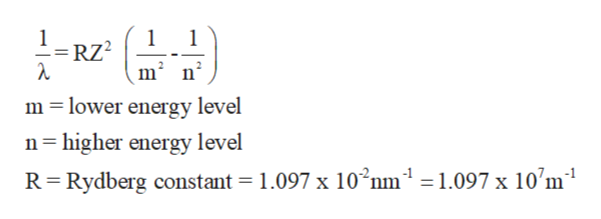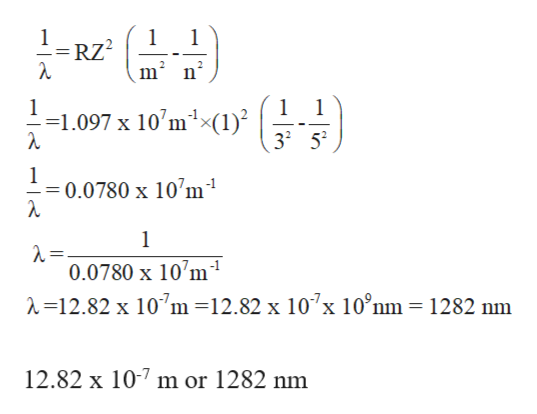# Calculate the wavelength of the photon emitted when an electron makes a transition from n=5to n=3. You can make use of the following constants:h=6.626×10−34J⋅sc=2.998×108m/s1 m=109nmExpress your answer to four significant figures and include the appropriate units. λλ =

Question
45 views

Calculate the wavelength of the photon emitted when an electron makes a transition from
n=5
to
n=3
. You can make use of the following constants:
h=6.626×
10
−34
J⋅s
c=2.998×
10
8
m/s
1 m=
10
9
nm
Express your answer to four significant figures and include the appropriate units.

λ
λ
=

check_circle

Step 1

Electromagnetic spectrum involves different radiations from the lowest to the highest frequency values. Some common radiations which are part of electromagnetic radiations are infrared radiation, visible light, ultraviolet radiation, X-rays, and gamma rays.

Step 2

The wavelength of radiation that involves in the transition of electron from one energy level to another can be calculated as:help_outlineImage Transcriptionclose1 1 RZ2 m n 1 m lower energy level n higher energy level R Rydberg constant = 1.097 x 10 nm1 = 1.097 x 10'm1 fullscreen
Step 3

Substitute the values to calculate the wavelength f...help_outlineImage Transcriptionclose1 RZ2 m2 n2 1 1.097 x 10 m(1) 1 1 32 52 1 0.0780 x 10'm1 1 0.0780 x 10'm =12.82 x 107m =12.82 x 107x 10°nm = 1282 nm 12.82 x 107 m or 1282 nm fullscreen

### Want to see the full answer?

See Solution

#### Want to see this answer and more?

Solutions are written by subject experts who are available 24/7. Questions are typically answered within 1 hour.*

See Solution
*Response times may vary by subject and question.
Tagged in

### Other